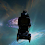### C C++ CODE : Gauss jordan method for finding inverse matrix

Working C C++  Source code program for Gauss jordan method for finding inverse matrix
``````/*************** Gauss Jordan method for inverse matrix ********************/
#include<iostream.h>
#include<conio.h>
int main()
{
int i,j,k,n;
float a={0},d;
clrscr();
cout<<"No of equations ? "; cin>>n;
cout<<"Read all coefficients of matrix with b matrix too "<<endl;
for(i=1;i<=n;i++)
for(j=1;j<=n;j++)
cin>>a[i][j];

for(i=1;i<=n;i++)
for(j=1;j<=2*n;j++)
if(j==(i+n))
a[i][j]=1;

/************** partial pivoting **************/
for(i=n;i>1;i--)
{
if(a[i-1]<a[i])
for(j=1;j<=n*2;j++)
{
d=a[i][j];
a[i][j]=a[i-1][j];
a[i-1][j]=d;
}
}
cout<<"pivoted output: "<<endl;
for(i=1;i<=n;i++)
{
for(j=1;j<=n*2;j++)
cout<<a[i][j]<<"    ";
cout<<endl;
}
/********** reducing to diagonal  matrix ***********/

for(i=1;i<=n;i++)
{
for(j=1;j<=n*2;j++)
if(j!=i)
{
d=a[j][i]/a[i][i];
for(k=1;k<=n*2;k++)
a[j][k]-=a[i][k]*d;
}
}
/************** reducing to unit matrix *************/
for(i=1;i<=n;i++)
{
d=a[i][i];
for(j=1;j<=n*2;j++)
a[i][j]=a[i][j]/d;
}

for(i=1;i<=n;i++)
{
for(j=n+1;j<=n*2;j++)
cout<<a[i][j]<<"    ";
cout<<endl;
}

getch();
return 0;
}

``````

1.correctly work
Thank you very much friend...........

2.why does the second for of reducing to diagonal matrix goes from j=1 to j<=n*2 ?

3.What if the value of a[i][i] = 0 in "reducing to diagonal matrix"?How to handle the "divide by zero" condition inthe calcualtion of "d"?

1.I have the same question.. Did you find any answer for that?

2.Thats why pivoting is done , to avoid dividing by zero.

4.Nice Work
--> Start Array indexing from i = 0;
--> Allocate Memory After value of n is known.

5.Thank you very much

6.I wonder what is the partial pivoting for?, I ran the code without it and just worked fine, so I am confuse about it.

Your Comment and Question will help to make this blog better...